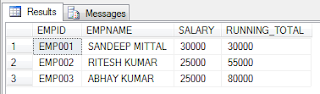## Feb 19, 2012## Running Total in Sql Server

This post is related with sharing my thoughts on calculating Running Total in Sql Server.

In this post I am sharing how running total can be calculated using recursive Common Table Expression (CTE).

NOTE: Solution would work with SQL Server 2005 onward

Example
Suppose we have Employee Table and we would calculate running total of Salary Column

Let's first create Employee Table and populate some data in it
```CREATE TABLE TBLEMPLOYEE (
EMPID        CHAR(6)
, EMPNAME    VARCHAR(50)
, SALARY    INT
)
INSERT INTO TBLEMPLOYEEINSERT INTO TBLEMPLOYEE
SELECT 'EMP001', 'SANDEEP MITTAL', 30000
UNION ALL SELECT 'EMP002', 'RITESH KUMAR', 25000
UNION ALL SELECT 'EMP003', 'ABHAY KUMAR', 25000
```

Now lets write script to calculate Running Total of Salary Column from the Employee Table using recursive CTE.
```DECLARE @TAB TABLE(
EMPID            CHAR(6)
, EMPNAME        VARCHAR(50)
, SALARY        INT
, RUNNING_TOTAL INT
)
INSERT INTO @TAB (EMPID, EMPNAME, SALARY)
SELECT  EMPID, EMPNAME, SALARY
FROM    TBLEMPLOYEE

DECLARE @RUNNING_TOTAL INT = 0
;WITH RUNNING_TOTAL AS (
SELECT    EMPID, EMPNAME, SALARY, RUNNING_TOTAL
FROM    @TAB
)
UPDATE RUNNING_TOTAL
SET  @RUNNING_TOTAL = RUNNING_TOTAL = @RUNNING_TOTAL + SALARY

SELECT * FROM @TAB```
OUTPUT

Refer related post to calculate cumulative sum using row_number,
Cumulative Sum in SQL Server

Refer related post for SQL Server 2012,
Cumulative Sum in SQL Server 2012

## Feb 5, 2012## Difference in Years, Months and Days in C#

In an earlier post, I have shared the function of calculating Date difference in Year(s), Month(s) and Day(s) in SQL Server.

Recently one of my friend had a requirement to calculate the same in ASP.Net, so I created a function to achieve the same in ASP.Net / C# and thought of posting the same on my blog.

Example:
Calculate Age in Years, Months and Days.

Solution:
Function DateDiffInYearMonthDay take two arguments of date datatype and returns the result in text format
Ex - 18 years, 2 months, 3 days

```private string DateDiffInYearMonthDay(DateTime fromDate, DateTime toDate)
{
int Years=0, Months=0, Days=0;
DateTime newDate;
newDate = fromDate;
while (newDate <= toDate)
{
Years++;
}
Years--;